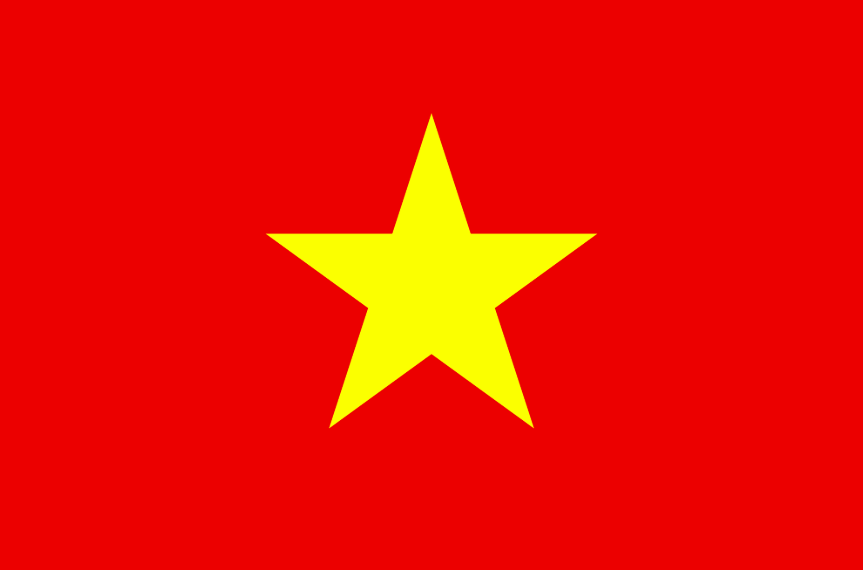Sunday, 27 May 2018

Pangarau in Ruma Waru

By discussing children's thoughts, children are clarifying their learning.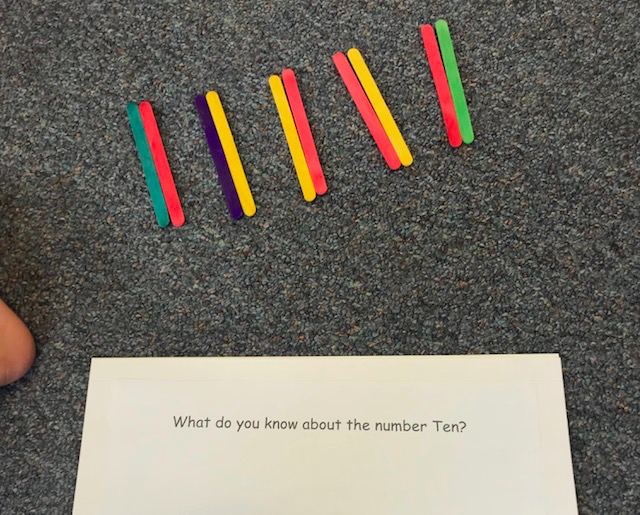We can use materials or draw pictures to solve word problems.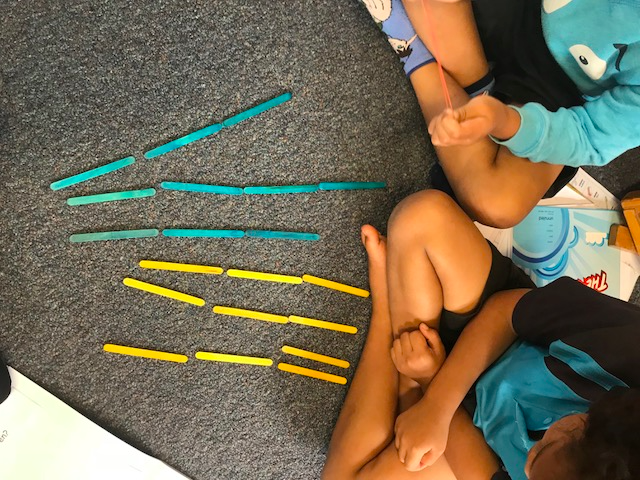3 + 3 + 4 = 10
3 + 3 + 3 + 1 = 10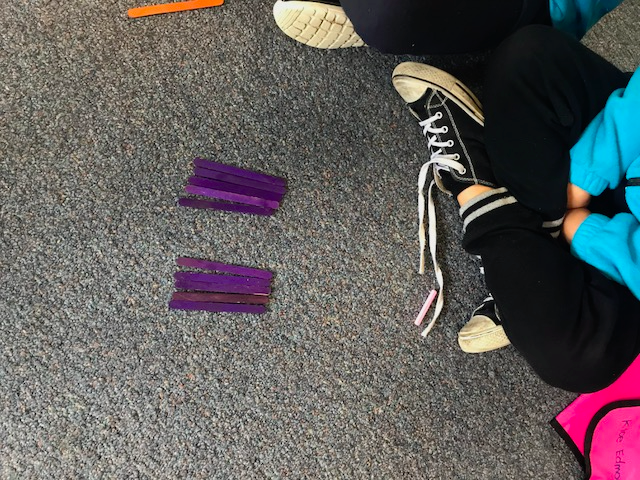5 + 5 = 106 + 4 =10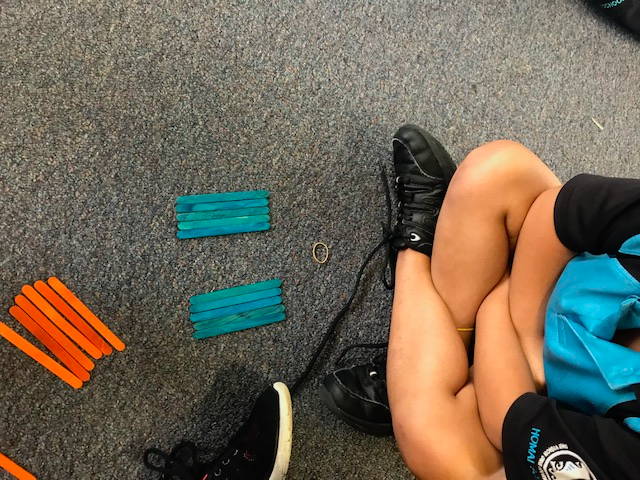5 + 5 = 10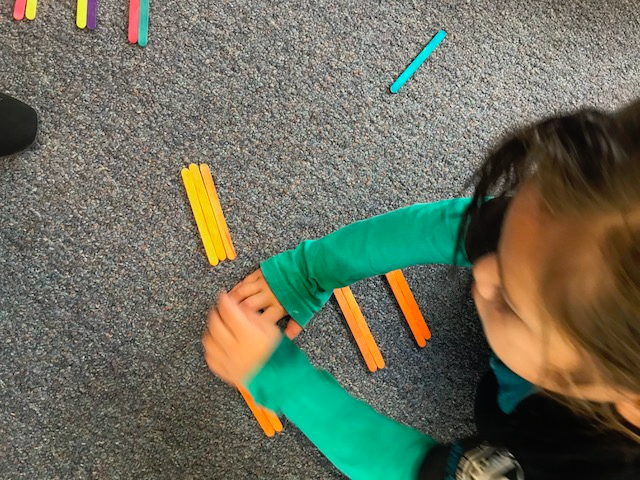3 + 3 + 2 + 2 = 10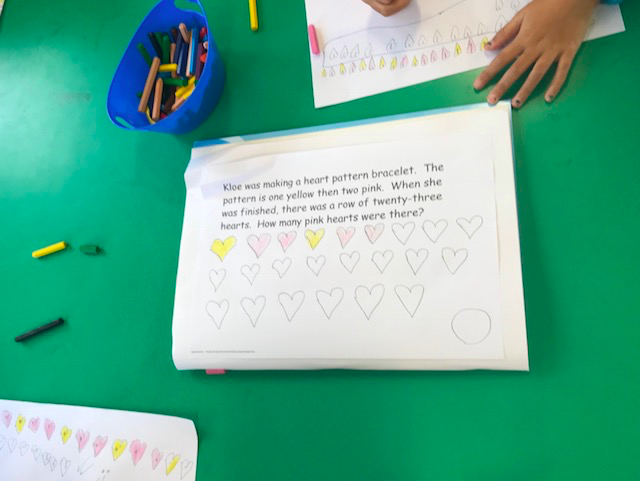Mrs Watson's Group working on patterns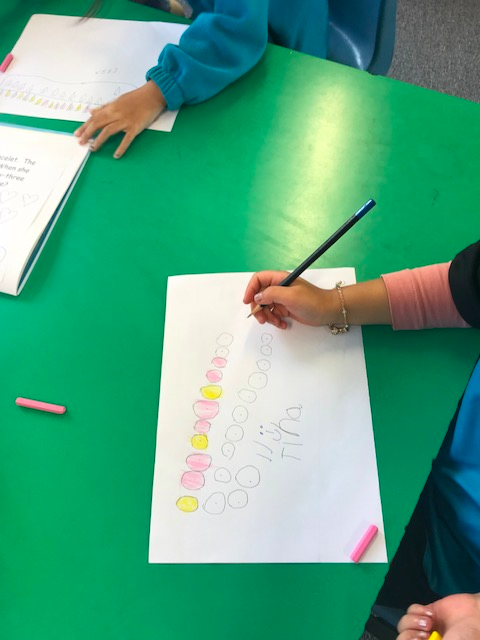Tina making sure that it's 1 yellow 2 pink